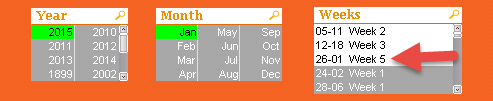# QlikView App Dev

Discussion Board for collaboration related to QlikView App Development.

Announcements
Customer & Partners, DEC. 9, 11 AM ET: Qlik Product & Strategy Roadmap Session: Data Analytics REGISTER NOW
cancel
Showing results for
Did you mean:Not applicable

## Weeks in current month

Hi

I have a listbox which shows me the Weekstart date - Weekend date(Mon-Sun) and the Week number of the current month

Problem is it shows me dates in the following month if the last day of the month is not on sundayI would like Week 5 to be 26 - 31 Week 5... how can I do this? I've been trying to crack this for a month nowscript:

Num(If( Len(Day(WeekStart("RE_DAY"))) >0, Day(WeekStart("RE_DAY")) ),'00')
&'-'&
Num(If( Len(Day(WeekEnd("RE_DAY")))>0, Day(WeekEnd("RE_DAY"))),'00')
&' Week '&
IF( ( week(WeekStart(RE_DAY)) - week(MonthStart(RE_DAY))+1) < 0, ( week(WeekStart(RE_DAY)) - week(MonthStart(RE_DAY))+1)+52, ( week(WeekStart(RE_DAY)) - week(MonthStart(RE_DAY))+1)) AS Weeks

1 Solution

Accepted SolutionsPartner

Here are all you need, you must only combine them.(StartDay, EndDay and WeekInMonth)

date(date('1.1.2015')+IterNo()) as Date,

Month(date('1.1.2015')+IterNo()) as Month,

Year(date('1.1.2015')+IterNo()) as Year,

Weekname(date('1.1.2015')+IterNo()) as Weekname,

WeekDay(date('1.1.2015')+IterNo()) as WeekDay,

if(month(weekstart(date('1.1.2015')+IterNo()))=month(date('1.1.2015')+IterNo()),day(weekstart(date('1.1.2015')+IterNo())),1) as StartDay,

if(month(weekend(date('1.1.2015')+IterNo()))=month(date('1.1.2015')+IterNo()),day(weekend(date('1.1.2015')+IterNo())),day(monthend(date('1.1.2015')+IterNo()))) as EndDay,

div(WeekDay(monthstart(date('1.1.2015')+IterNo()))+day(date('1.1.2015')+IterNo())-1,7)+1 as WeekInMonth

AutoGenerate(1) while IterNo() < 600;

7 RepliesPartner

Want you have "26-31 Week 5" in Jan and "01-01 Week 5 1" in Feb?Not applicable
Author

That is correct, and if the last day of the month is on a Monday, I want it to be "31-31 Week 5" then "01-06 Week 1"
in the next month.Partner

Here are all you need, you must only combine them.(StartDay, EndDay and WeekInMonth)

date(date('1.1.2015')+IterNo()) as Date,

Month(date('1.1.2015')+IterNo()) as Month,

Year(date('1.1.2015')+IterNo()) as Year,

Weekname(date('1.1.2015')+IterNo()) as Weekname,

WeekDay(date('1.1.2015')+IterNo()) as WeekDay,

if(month(weekstart(date('1.1.2015')+IterNo()))=month(date('1.1.2015')+IterNo()),day(weekstart(date('1.1.2015')+IterNo())),1) as StartDay,

if(month(weekend(date('1.1.2015')+IterNo()))=month(date('1.1.2015')+IterNo()),day(weekend(date('1.1.2015')+IterNo())),day(monthend(date('1.1.2015')+IterNo()))) as EndDay,

div(WeekDay(monthstart(date('1.1.2015')+IterNo()))+day(date('1.1.2015')+IterNo())-1,7)+1 as WeekInMonth

AutoGenerate(1) while IterNo() < 600;Partner

Here the fileSpecialist II

My effort;

if(Month(WeekEnd(RE_DAY))>Month(RE_DAY),
round(Day(WeekStart(RE_DAY))/7)+1,
round(Day(WeekEnd(RE_DAY))/7)) AS Week

Which only works for Jan ... d'oh.Partner

You can also use.

day(RangeMax(weekstart(DATE),monthstart(DATE))) as StartDay,

day(RangeMin(weekend(DATE),monthend(DATE))) as EndDayNot applicable
Author

Hi Jonas

Thanks a lot man. You are very good at this hey...wow!Community Browser